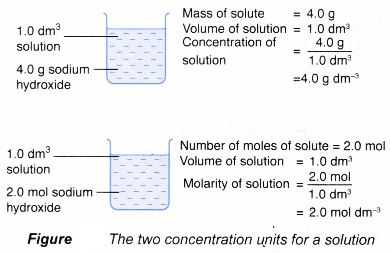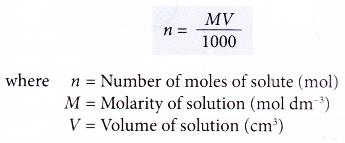# How to calculate concentration of acids and alkalis?

## How to calculate concentration of acids and alkalis?

Concentrations of Acids and Alkalis

1. A solution is a mixture formed by dissolving a solute in a solvent.
Solute + solvent → solution
For example, a sugar solution is prepared by dissolving sugar (solute) in water (solvent).
2. By dissolving varying amounts of sugar in a fixed volume of water, sugar solutions of different concentrations are obtained.
3. Hence, the concentration of a solution refers to the quantity of solute in a given volume of solution which is usually 1 dm3 of solution.
4. The quantity of solute can be measured in grams or moles.
5. Therefore, concentration of a solution can be expressed in two ways:
(a) mass of solute in grams in 1 dm3 of solution
(b) number of moles of solute in 1 dm3 of solution
6. The concentration expressed in moles per dm3 is called molarity or molar concentration.
Molarity is the number of moles of solute present in 1 dm3 of solution.7. Figure shows the two concentration units for a solution.### Calculating concentration in g dm-3

1. 4.0 g of sodium hydroxide, NaOH is dissolved in enough water to make 200 cm3 of solution.
What is the concentration of the solution in g per dm3?
Solution:2. A 400 cm3 salt solution was evaporated to dryness and 18.0 g of sodium chloride, NaCl, was left behind. Calculate the concentration of the salt solution in g dm-3.
Solution:3. Concentrated phosphoric acid, H3PO4 was added slowly to water to produce 5.0 dm3 of diluted acid solution. 100 g of pure acid was present in the solution. What is the concentration of the acid in g dm-3.
Solution:### Calculating concentration in mol dm-3

1. What is the molarity of a solution prepared by dissolving 0.15 mole of ammonia in enough distilled water to make 250 cm3 of solution?
Solution:2. Copper(II) oxide reacts with dilute sulphuric acid to produce 1.2 moles of copper(II) sulphate, CuSO4. The final volume of the salt solution is 1.8 dm3. What is the molar concentration of the salt solution?
Solution:### Conversion of units of concentration

The equations below show the interconversion between the two units of concentrations:1. A 200 cm3 solution was found to contain 24.5 g of dissolved sulphuric acid. What is the molarity of the acid solution?
[Relative atomic mass: H, 1; O, 16; S, 32]
Solution:2. An alkaline solution of potassium hydroxide, KOH has a concentration of 2.8 g dm-3. Calculate the molarity of the solution.
[Relative atomic mass: H, 1; O, 16; K, 39]
Solution:### Calculating the amount of solute

The number of moles of a solute in a given volume of solution can be calculated using the following equation.1. Calculate the mass of hydrochloric acid in 300 cm3 of 2.0 mol dm-3 hydrochloric acid.
[Relative atomic mass: H, 1; Cl, 35.5]
Solution:2. A student pipetted 25.0 cm3 of sodium hydroxide solution into a conical flask. The concentration of the alkali is 1.20 mol dm-3. Calculate the number of moles of sodium hydroxide in the flask.
Solution:3. Calculate the number of moles of hydrogen ions in 500 cm3 of 1.0 mol dm3 sulphuric acid.
Solution: